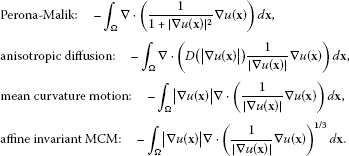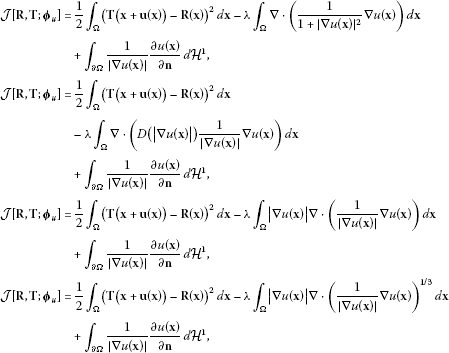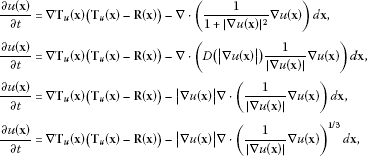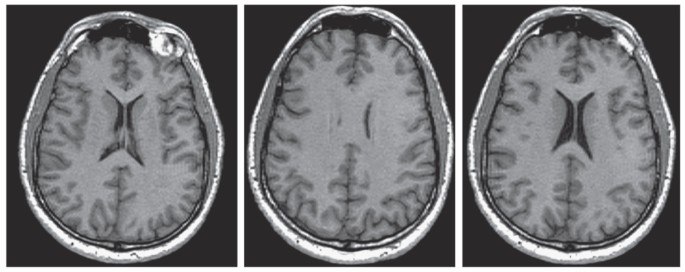Skip to main content

# Curvature-driven diffusion-based mathematical image registration models

## Abstract

This paper introduces several mathematical image registration models. Image registration, an ill-posed optimization problem, is formulated as the minimization of the sum of an image similarity metric and a regularization term. Curvature-driven diffusion-based techniques, in particular Perona-Malik, anisotropic diffusion, mean curvature motion (MCM), affine invariant MCM (AIMCM), are employed as a regularization term in this optimal control formulation. Adopting the steepest-descent marching with an artificial time t, Euler-Lagrange (EL) equations with homogeneous Neumann boundary conditions are obtained. These EL equations are approximately solved by the explicit Petrov-Galerkin scheme. The method is applied to the registration of brain MR images of size $257×257$. Computational results indicate that all these regularization terms produce similarly good registration quality but that the cost associated with the AIMCM approach is, on average, less than that for the others.

MSC:68U10, 65D18, 65J05, 97N40.

## 1 Introduction

The purpose of image registration is to align two or more images of the same scene obtained at different times, perspectives or sensors such as MRI, X-ray, CT, PET, SPECT, and tomography. Given a reference image $R(x)$ and a template image $T(x)$, the main idea behind an image registration paradigm is to find a reasonable transformation such that the transformed image becomes similar to the reference image. Image registration has a broad range of applications such as object or motion tracking, detecting tumors, image fusion among many others; see, for example, . Image registration is a significant and challenging subject which usually involves high storage requirements, high CPU costs and mostly deals with noisy, distorted, and occluded data. In literature several different types of image registration techniques (see, for instance, [2, 3] and references therein) were developed. Each of these algorithms was generated based on a specific application, disease or image modality. There is still no general image registration technique which could be used in every sort of data. Based on these facts, finding fast and efficient image registration techniques is a quite useful and still significantly important area of research.

In this paper, we express the image registration algorithm as a variational optimization problem which consists of the sum of a similarity measure and a regularization term. Our method incorporates $L 2$-norm sense similarity measures with several different curvature-driven diffusion-based regularization terms such as anisotropic diffusion, mean curvature motion (MCM), and affine invariant MCM. We are mostly motivated by the efficient performance of these regularization terms in image denoising and restoration problems (for instance,  applies these regularization terms to image processing problems). It is significantly important to explore the utility of these geometrically derived regularization terms in the field of image deformation.

Organization of the paper is as follows. In the Section 2, we present an optimal control formulation of the image registration problem. We use the sum of squared differences in the $L 2$-norm sense as the similarity measure and introduce four different regularization terms. In the same section, we solve the existing optimization problem in a systematic manner by the techniques in variational calculus such as gradient-descent based methods. Computational results given in the last section indicate that new regularization terms provide fast, stable, and efficient image registration models.

## 2 Optimal control formulation of image registration problem

The state-of-the-art image registration problem can be expressed in the following way. Assume that both template T and reference R images are defined on the same domain Ω. Then the image registration problem can be formulated as the optimization problem

$min ϕ ∈ Γ J[R,T; ϕ u ]$
(1)

for the functional

$J[R,T; ϕ u ]= C sim [R,T; ϕ u ]+λ C reg [u],$
(2)

where $C sim [R,T; ϕ u ]$ denotes a similarity measure between the template image T and the reference image R, $ϕ u (x):=x+u(x)$ is the deformation field, u is the displacement field, Γ is the set of all possible admissible transformations, $C reg [u]$ is a regularization term, and λ is a regularization constant. Because reference and template images are obtained from different distances, angles, times, and sometimes even by different individuals, a deformation field may occur between these images. A deformation field is a vector field that maps pixels of the reference image to the corresponding ones of the template image. One of the major goals of this paper is to compute the deformation field in a systematic way.

We choose the $L 2$-norm type similarity measure defined as

$C sim [ R ( x ) , T ( x ) ; ϕ ( x ) ] = 1 2 ∫ Ω ( T ( x + u ( x ) ) − R ( x ) ) 2 dx.$
(3)

This similarity measure is often referred to as the ‘sum of squared differences’ (SSD) measure. Note that other similarity measures can be selected depending on the problem. We choose the similarity measure (3) due to its well-known effectiveness (for example, see ), the convenience in computations, and easy adaptation of the regularization terms in numerical computations.

Image registration is an ill-posed optimal control problem. In order to overcome the ill-posedness of the optimization problem (1) and to assure smooth solutions, we introduce additional regularization terms. The main idea behind adding a regularization term is to smoothen the problem with respect to both the functional and the solution so that well-posedness is assured and efficient computational methods can be developed to determine minimizers. In this paper, we use some curvature-driven diffusion-based techniques, in particular Perona-Malik, anisotropic diffusion, mean curvature motion, affine invariant mean curvature motion regularization terms. Next, we present each of these regularization terms:For the convenience of computations, we define $T u (x):=To ϕ u (x)=T(x+u(x))$. Writing these regularization terms in the functional (2) and taking into account the boundary conditions, for each of these regularization terms, we can express the functional (2) aswhere n denotes the outer normal along the boundary Ω, and $d H 1$ is the one-dimensional Hausdorff measure supported on Ω. Here, the divergence in the regularization terms is understood in the distributional meaning. In  we studied the same optimal control formulation of the image registration problem with different regularization terms and showed the existence and uniqueness of this optimization problem using appropriate theorems. Similar sorts of optimal control problems with their computational solutions might be seen in [5, 6].

From these equations, by adopting the steepest-descent marching with an artificial time t, we getwith homogeneous Neumann boundary conditions along Ω.

Sethian  developed a good number of efficient schemes for the numerical solution of these types of equations, some of which are the fast marching scheme, the explicit positive-coefficient scheme, the explicit Petrov-Galerkin scheme, and some other schemes for so-called curvature type flows. Aubert et al.  also developed some efficient numerical solution methods for these sorts of equations. We adopted the explicit Petrov-Galerkin scheme and implemented the corresponding algorithm in Matlab computer algebra system. Next section shows the experimental results.

## Computational results

In this section, we present an illustrative example where we demonstrate the registration of brain MR images in the size of $257×257$ as an application of the proposed models. The template, reference, and registered images with AIMCM are shown in Figure 1. Computational examples indicate that all regularization terms produce similarly good registration quality, but the cost associated with affine invariant MCM approach is, on average, less than that for others. It could be said that the quality of the registration is correlated with the size of the SSD in an opposite way. In other words, the smaller the size of SSD, the better registered images. Table 1 shows the changes in SSD for each model used versus the number of iterations. The duration of the registration with the AIMCM and MCM models is almost 1 minute and the one with Perona-Malik and anisotropic diffusion (AD) is almost 2.5 minutes. We applied the presented method to some other brain MR images and obtained similar results. Because the registered image is similar to other regularization terms, we omit to present them inhere for the sake of brevity.Figure 1

## Concluding remarks

Medical image registration is quite an important and challenging branch of image processing world. It has broad applications in medical and non-medical imaging.

In this paper, we presented a number of curvature-driven diffusion-based image registration techniques. Our method incorporates the sum of a squared differences similarity measure with several different regularization terms such as Perona-Malik, anisotropic diffusion, mean curvature motion, and affine invariant MCM. To the best of our knowledge, nobody in the literature associated these regularization terms with the SSD similarity measure and applied them to the nonrigid registration of medical images. By using gradient-descent based optimization techniques, we solve the existing optimization problem and obtain the corresponding optimality system in a systematic manner. Exploiting the explicit Petrov-Galerkin scheme, we implemented the corresponding algorithm in Matlab.

In future work, we will investigate the applications of these image registration techniques to the registration of noisy and blurred images. Furthermore, we plan to compare the strength of these registration techniques with some well-known image registration methods in terms of speed, quality, and effectiveness in detail.

## References

1. 1.

Akinlar, MA: A new method for non-rigid registration of 3D images. PhD thesis, The University of Texas at Arlington (2009)

2. 2.

Akinlar MA, Celenk M: Quality assessment for an image registration. Int. J. Contemp. Math. Sci. 2011, 6(30):1483–1490.

3. 3.

Akinlar MA, Kurulay M, Secer A, Bayram M: Efficient variational approaches for deformable registration of images. Abstr. Appl. Anal. 2012. doi:10.1155/2012/704567

4. 4.

Scherzer O, Grasmair M, Grossauer H, Haltmeier M, Lenzen F: Variational Methods in Imaging. Springer, Berlin; 2009.

5. 5.

Zheng G, Ma B: A time optimal control problem of some linear switching controlled ordinary differential equations. Adv. Differ. Equ. 2012., 2012: Article ID 52. doi:10.1186/1687–1847–2012–52

6. 6.

Chen P, Li Y, Fan H: Existence of strong solutions for a class of semilinear evolution equations with nonlocal initial conditions. Adv. Differ. Equ. 2012., 2012: Article ID 79. doi:10.1186/1687–1847–2012–79

7. 7.

Sethian JA: Level Set Methods and Fast Marching Methods. 9th edition. Cambridge University Press, Cambridge; 2008.

8. 8.

Aubert G, Kornprobst P: Mathematical Problems in Image Processing. 2nd edition. Springer, Berlin; 2006.

Download references

## Author information

Authors

### Corresponding author

Correspondence to Aydin Secer.

## Additional information

### Competing interests

The authors declare that they have no competing interests.

### Authors’ contributions

Before this paper the authors made a sequence of scientific discussions and exchanged research ideas about generating new image registration models. Finally MAA and MC suggested to combine curvature driven diffusion based regularization terms with image registration problems. Both authors also point to use the sum of square differences as a similarity metric in the optimal control formulation of the image registration problem. MK and AS solved the optimization problem mathematically and both of these authors implemented the algorithm in Matlab programming language. MAA and MC suggested to use MR images in the implementation. The authors interpreted the results all together.

## Authors’ original submitted files for images

Below are the links to the authors’ original submitted files for images.

## Rights and permissions

Reprints and Permissions

## About this article

### Cite this article

Akinlar, M.A., Kurulay, M., Secer, A. et al. Curvature-driven diffusion-based mathematical image registration models. Adv Differ Equ 2012, 193 (2012). https://doi.org/10.1186/1687-1847-2012-193

Download citation

• Received:

• Accepted:

• Published:

### Keywords

• sum of squared differences
• inverse problems
• computational modeling
• Petrov-Galerkin scheme
• image registration